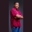Related Tags

line number
swift
source code
communitycreator

# How to print the line number of a Swift source codeTheodore Kelechukwu Onyejiaku

### Overview

In a source code, each line is referred to as a line number. We can write our code and also print the line number of each line of code.

### Syntax

print(#line)
Syntax to print line numbers of a source code

### Parameters

#line: This represents each line number in the source code.

### Return value

It returns the line number of each source code.

### Example

import Swift

// create some variables
var num1 = 34;
var num2 = 50;

print("This is line: ", #line)
print("The sum of ", num1, " and ", num2, "is and line number is ", #line)
Get the line number of a source code

### Explanation

• Lines 4 and 5: We create some variables and initialize them.
• Line 7: We print the line number.
• Line 8: We print the sum of the variables we created and print the line numbers.

RELATED TAGS

line number
swift
source code
communitycreator

CONTRIBUTORTheodore Kelechukwu Onyejiaku
RELATED COURSES

View all Courses

Keep Exploring

Learn in-demand tech skills in half the time